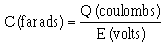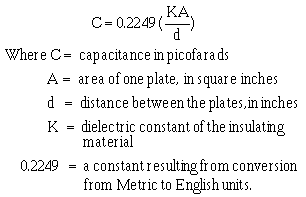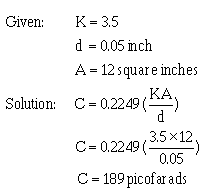Custom SearchCapacitance is measured in units called FARADS. A one-farad capacitor stores one coulomb (a unit of charge (Q) equal to 6.28 X 1018 electrons) of charge when a potential of 1 volt is applied across the terminals of the capacitor. This can be expressed by the formula:The farad is a very large unit of measurement of capacitance. For convenience, the microfarad (abbreviated mF) or the picofarad (abbreviated pF) is used. One (1.0) microfarad is equal to 0.000001 farad or 1 X 10-6 farad, and 1.0 picofarad is equal to 0.000000000001 farad or 1.0 X 10-12 farad. Capacitance is a physical property of the capacitor and does not depend on circuit characteristics of voltage, current, and resistance. A given capacitor always has the same value of capacitance (farads) in one circuit as in any other circuit in which it is connected.

FACTORS AFFECTING THE VALUE OF CAPACITANCE

• The value of capacitance of a capacitor depends on three factors:
• The area of the plates.
• The distance between the plates.
• The dielectric constant of the material between the plates.

PLATE AREA affects the value of capacitance in the same manner that the size of a container affects the amount of water that can be held by the container. A capacitor with the large plate area can store more charges than a capacitor with a small plate area. Simply stated, "the larger the plate area, the larger the capacitance".

The second factor affecting capacitance is the DISTANCE BETWEEN THE PLATES. Electrostatic lines of force are strongest when the charged particles that create them are close together. When the charged particles are moved further apart, the lines of force weaken, and the ability to store a charge decreases.

The third factor affecting capacitance is the DIELECTRIC CONSTANT of the insulating material between the plates of a capacitor. The various insulating materials used as the dielectric in a capacitor differ in their ability to respond to (pass) electrostatic lines of force. A dielectric material, or insulator, is rated as to its ability to respond to electrostatic lines of force in terms of a figure called the DIELECTRIC CONSTANT. A dielectric material with a high dielectric constant is a better insulator than a dielectric material with a low dielectric constant. Dielectric constants for some common materials are given in the following list:

 Material Constant Vacuum 1.0000 Air 1.0006 Paraffin paper 3.5 Glass 5 to 10 Mica 3 to 6 Rubber 2.5 to 35 Wood 2.5 to 8 Glycerine (15C) 56 Petroleum 2 Pure Water 81

Notice the dielectric constant for a vacuum. Since a vacuum is the standard of reference, it is assigned a constant of one. The dielectric constants of all materials are compared to that of a vacuum. Since the dielectric constant of air has been determined to be approximately the same as that of a vacuum, the dielectric constant of AIR is also considered to be equal to one.

The formula used to compute the value of capacitance is:Example: Find the capacitance of a parallel plate capacitor with paraffin paper as the dielectric.By examining the above formula you can see that capacitance varies directly as the dielectric constant and the area of the capacitor plates, and inversely as the distance between the plates.

Q.7 State three factors that affect the capacitance of a capacitor.
Q.8 A parallel plate capacitor has the following values: K = 81, d = .025 inches, A = 6 square inches. What is the capacitance of the capacitor?

VOLTAGE RATING OF CAPACITORS

In selecting or substituting a capacitor for use, consideration must be given to (1) the value of capacitance desired and (2) the amount of voltage to be applied across the capacitor. If the voltage applied across the capacitor is too great, the dielectric will break down and arcing will occur between the capacitor plates. When this happens the capacitor becomes a short-circuit and the flow of direct current through it can cause damage to other electronic parts. Each capacitor has a voltage rating (a working voltage) that should not be exceeded.

The working voltage of the capacitor is the maximum voltage that can be steadily applied without danger of breaking down the dielectric. The working voltage depends on the type of material used as the dielectric and on the thickness of the dialectic. (A high-voltage capacitor that has a thick dielectric must have a relatively large plate area in order to have the same capacitance as a similar low-voltage capacitor having a thin dielectric.) The working voltage also depends on the applied frequency because the losses, and the resultant heating effect, increase as the frequency increases.

A capacitor with a voltage rating of 500 volts dc cannot be safely subjected to an alternating voltage or a pulsating direct voltage having an effective value of 500 volts. Since an alternating voltage of 500 volts (rms) has a peak value of 707 volts, a capacitor to which it is applied should have a working voltage of at least 750 volts. In practice, a capacitor should be selected so that its working voltage is at least 50 percent greater than the highest effective voltage to be applied to it.

CAPACITOR LOSSES

Power loss in a capacitor may be attributed to dielectric hysteresis and dielectric leakage. Dielectric hysteresis may be defined as an effect in a dielectric material similar to the hysteresis found in a magnetic material. It is the result of changes in orientation of electron orbits in the dielectric because of the rapid reversals of the polarity of the line voltage. The amount of power loss due to dielectric hysteresis depends upon the type of dielectric used. A vacuum dielectric has the smallest power loss.

Dielectric leakage occurs in a capacitor as the result of LEAKAGE CURRENT through the dielectric. Normally it is assumed that the dielectric will effectively prevent the flow of current through the capacitor. Although the resistance of the dielectric is extremely high, a minute amount of current does flow. Ordinarily this current is so small that for all practical purposes it is ignored. However, if the leakage through the dielectric is abnormally high, there will be a rapid loss of charge and an overheating of the capacitor.

The power loss of a capacitor is determined by loss in the dielectric. If the loss is negligible and the capacitor returns the total charge to the circuit, it is considered to be a perfect capacitor with a power loss of zero.

Q.9 Name two types of power losses associated with a capacitor.
Q.10 Define the term "working voltage" of a capacitor. What should be the working voltage of a capacitor in a circuit that is operating at 600 volts?Integrated Publishing, Inc. - A (SDVOSB) Service Disabled Veteran Owned Small Business